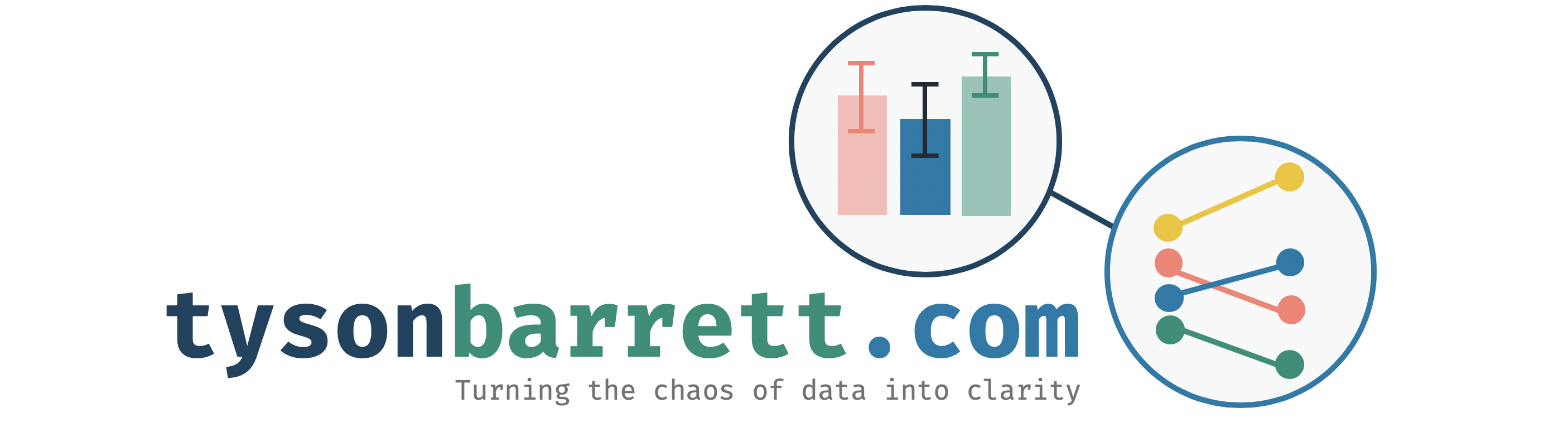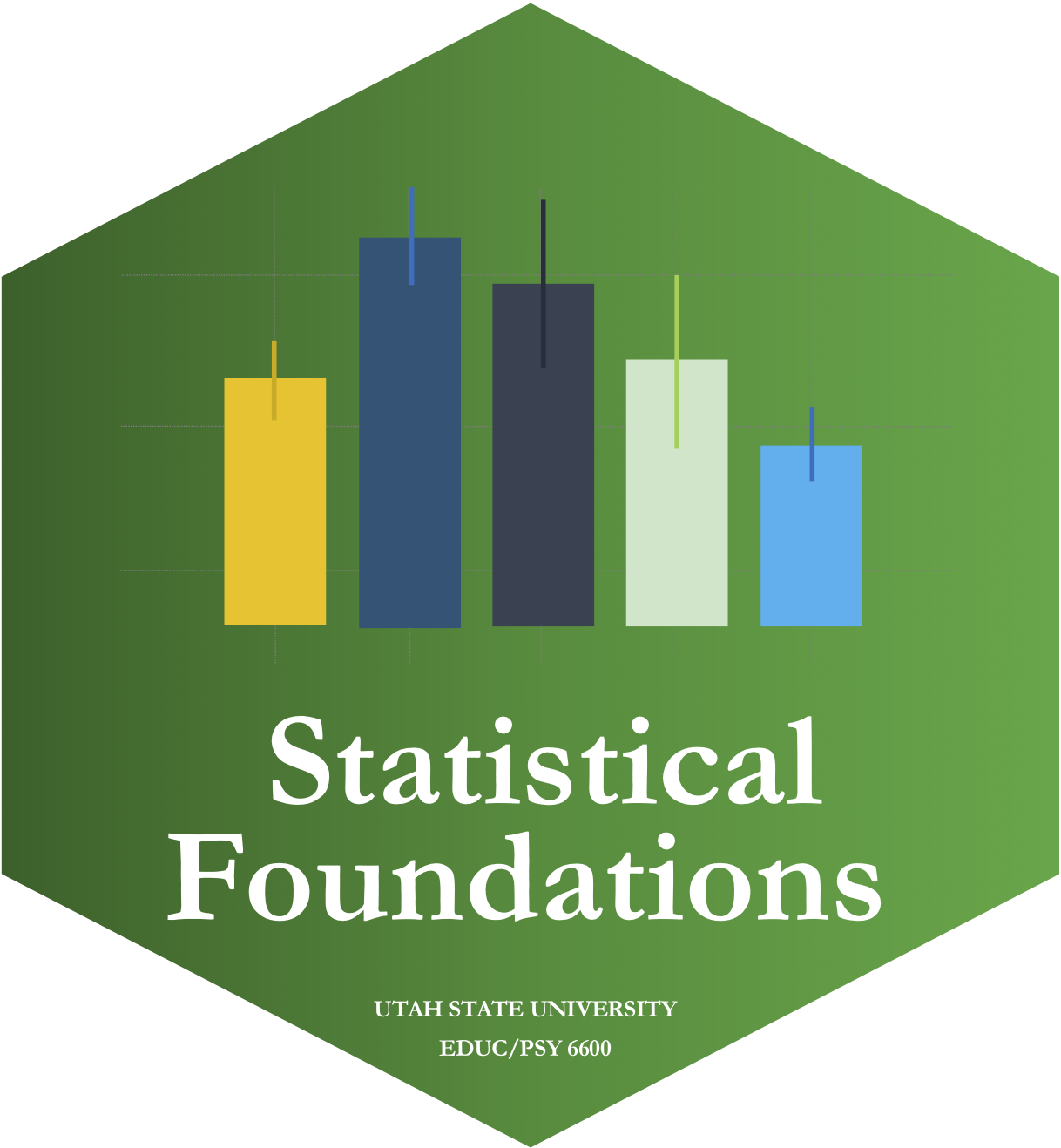### Statistical Foundations

This course is an introduction to statistics at a graduate level (generally PhD students take this course in preparation for further statistical training). Discussion includes topics such as data visualization, exploratory data analysis, and statistical tests (t-tests, ANOVA). This course uses R and RStudio for all data analyses.

Syllabus

## Resources

• A PDF with all the formulas we use in the class.
• A PDF with all the tables we use in the class.

## Final Project

The final project is a short article that includes one of the main methods from 3 of the 4 units. For example, an article that uses an exploratory plot (Unit 1), a 1-way ANOVA (Unit 2), and a repeated measures ANOVA (Unit 3) would work. The article should have a very short introduction (one paragraph that introduces the idea and research questions), a thorough methods section showing that you understand how to use the methods in practice (including testing for assumptions), a thorough results section showing that you can interpret the resulting output correctly, and a very short discussion (one paragraph stating how the results apply to your general research questions from the introduction).

• Should be less than 10 pages (no minimum limit)
• Should include (as a separate file or appendix) the code used to produce the results (practice using open science!)